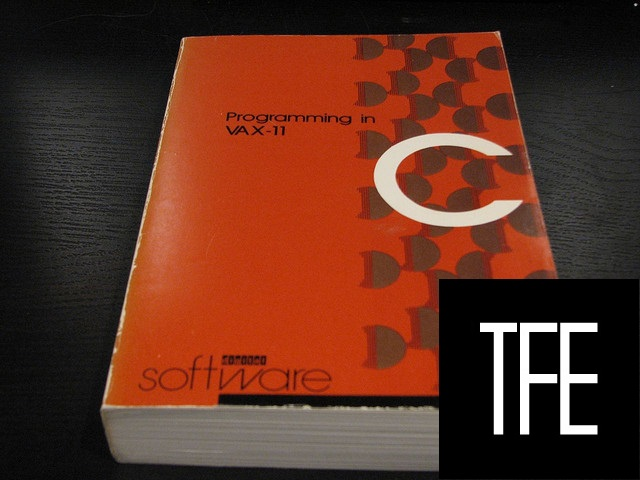# With C arrays, why is it the case that a == 5[a]?As Joel points out in Stack Overflow podcast #34, in C Programming Language (aka: K & R), there is mention of this property of arrays in C: a == 5[a]

Joel says that it’s because of pointer arithmetic but I still don’t understand. Why does `a == 5[a]` ?

Dinah

The C standard defines the `[]` operator as follows:

`a[b] == *(a + b)`

Therefore `a` will evaluate to:

``*(a + 5)``

and `5[a]` will evaluate to:

``*(5 + a)``

and from elementary school math we know those are equal.

This is the direct artifact of arrays behaving as pointers, “`a`” is a memory address. “`a`” is the value that’s 5 elements further from “`a`“. The address of this element is “`a + 5`“. This is equal to offset “`a`” from “`5`” elements at the beginning of the address space (`5 + a`).

Stack Overflow